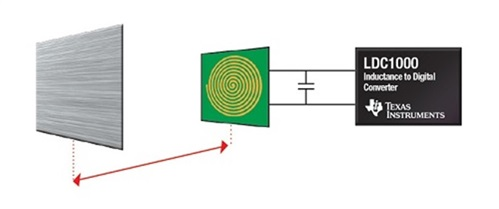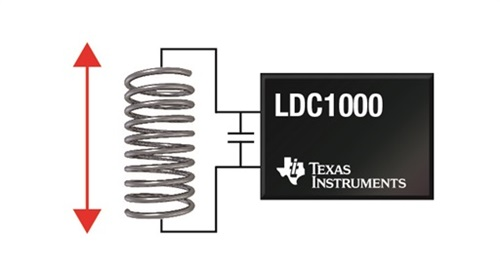# Inductive sensing: Should I measure L, RP or both?

When devices offer different types of measurement capabilities, it’s important for designers to consider which measurement is best suited for their use case. Some inductive sensing solutions, like TI’s LDC1000 inductance-to-digital converter (LDC), have two measurement capabilities:

• RP-measurement: The LDC measures the equivalent parallel impedance of the sensor at its resonant frequency by measuring the energy loss of the sensor due to magnetic coupling with a conductive target.
• Inductance (L)-measurement: The LDC measures the resonant frequency of the sensor, which is a function of sensor inductance, also influenced by magnetic coupling with a conductive target.

Some LDCs, such as the LDC1000, even offer both measurement capabilities.

Having these two measurement capabilities leads to a few questions:

• Do you always need to measure both parameters?
• If you only need one, which one should you choose?

Let's compare the two measurement types and explore a few different use cases.

Sensing range and precision

The maximum sensing range is similar for L- and RP-measurements and depends primarily on coil diameter, resolution of the LDC and device configuration. A useful rule of thumb for precision applications is that an LDC requires a coil diameter of at least twice the maximum sensing range (for example, we would need a 20 mm diameter coil to measure a target distance up to 10 mm). This applies to both L-measurements and RP-measurements.Figure 1. Axial position sensing

Reference clock input

Inductance is measured by determining the oscillation frequency shift when the conductive target approaches the sensor coil. As a result, it requires an accurate and stable reference clock. RP-measurements do not rely on an accurate reference clock and the LDC1000 can perform RP-measurements without an external reference clock. This is an advantage in situations where a reference clock is not available, or where number of wires between the LDC and the microcontroller must be minimized.

Temperature

Temperature drift in L-measurements is small compared to the temperature drift in RP-measurements. When using a high-Q sensor, which helps minimize temperature effects, temperature compensation in L-measurement applications is typically only required when you need very high precision over a wide system temperature range.

On the other hand, the resistivity of any metal has a known but significant temperature coefficient, which becomes relevant in RP-measurements. For example, the resistivity of copper changes by 3900 ppm/°C, aluminum by 3900 ppm/°C and iron by 5000 ppm/°C. To account for the change in resistivity, temperature compensation is typically required for most applications that employ RP-measurement.

Spring compression applications

Compressing, extending or twisting a spring changes its length, diameter and/or number of turns, which in turn changes the spring inductance. Therefore, measuring inductance directly, rather than RP, is the obvious choice for this application.Figure 2. Spring compression measurement

Metal composition applications

Inductive sensing can be used to differentiate between different types of metals. In such applications, an L-measurement provides information on the permeability (μ) of the metal, because the inductance of the system is greater with greater μ of the metal. By contrast, an RP-measurement provides information on the resistivity (ρ) of the metal.

As eddy currents flow through the conductive target, the induced electric energy is dissipated based on the value of ρ. This is indicated by a change in RP. By generating a table of inductance and RP at a fixed distance from the coil, we can identify different metal alloys. To detect metal composition, we need to measure RP and L simultaneously.

Metal choice

Most metal types can be equally well-measured with L or RP. However, there are some magnetic materials where the L response at certain frequencies is significantly smaller than the RP response. For those materials, RP is a more appropriate choice. We will cover this topic in more detail in an upcoming blog post.

Which measurement approach will you use?

For most applications, you may prefer the reduced system design complexity of L-measurements due to lower temperature effects. There are two exceptions, in which RP measurements are  required: systems in which no accurate reference clock is available and selected designs use magnetic materials as a target. And metal composition detection demands measuring both parameters simultaneously.

Additional resources: